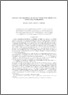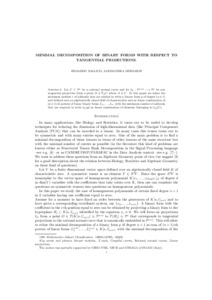# Minimal decomposition of binary forms with respect to tangential projections.

Ballico, Edoardo ; Bernardi, Alessandra (2010) Minimal decomposition of binary forms with respect to tangential projections. [Preprint]
Full text available as:Preview PDF Download (193kB) | Preview

## Abstract

Let $C\subset \PP n$ be a rational normal curve and let $\ell_O:\PP {n+1}\dashrightarrow \PP n$ be any tangential projection form a point $O\in T_AC$ where $A\in C$. In this paper we relate the minimum number $r$ of addenda that are needed to write a binary form $p$ of degree $(n+1)$ and defined over an algebraically closed field of characteristic zero as linear combination of $(n+1)$-th powers of linear binary forms $L_1, \ldots , L_r$, with the minimum number of addenda that are required to write $\ell_O(p)$ as linear combination of elements belonging to $\ell_O(C)$.

Abstract
Document type
Preprint
Creators
CreatorsAffiliationORCID
Ballico, Edoardo
Bernardi, Alessandra
Keywords
Secant varieties, $X$-rank, Cuspidal curves, Rational normal curves, Linear projections.
Subjects
DOI
Deposit date
19 Jul 2010 10:00